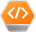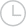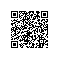# 如何将matlab代码转换成python代码?(-问答-阿里云开发者社区-阿里云

## 如何将matlab代码转换成python代码?(kun坤2019-12-25 21:47:271237

``````function rao1()
clc
clear all
pop = 10;                                % Population size
var = 30;    % Number of design variables
maxFes = 30000;                 % Maximum functions evaluation
maxGen = floor(maxFes/pop);     % Maximum number of iterations
mini = -100*ones(1,var);
maxi = 100*ones(1,var);
[row,var] = size(mini);
x  = zeros(pop,var);
for i=1:var
x(:,i) = mini(i)+(maxi(i)-mini(i))*rand(pop,1);
end
f = objective(x);
gen=1;
while(gen <= maxGen)
xnew = updatepopulation(x,f);
xnew = trimr(mini,maxi,xnew);
fnew = objective(xnew);
for i=1:pop
if(fnew(i)<f(i))
x(i,:) = xnew(i,:);
f(i) = fnew(i);
end
end
disp(['Iteration No. = ',num2str(gen)])
disp('%%%%%%%% Final population %%%%%%%%%')
disp([x,f])
fnew = [];
xnew = [];
[fopt(gen),ind] = min(f);
xopt(gen,:)= x(ind,:);
gen = gen+1;
end
[val,ind] = min(fopt);
Fes = pop*ind;
disp(['Optimum value = ',num2str(val,10)])
end
%%The objective function is given below.
function [f] = objective(x)
[r,c]=size(x);
for i=1:r
y=0;
for j=1:c
y=y+(x(i,j))^2;         % Sphere function
end
z(i)=y;
end
f=z';
end
function [xnew] = updatepopulation(x,f)
[row,col]=size(x);
[t,tindex]=min(f);
Best=x(tindex,:);
[w,windex]=max(f);
worst=x(windex,:);
xnew=zeros(row,col);
for i=1:row
for j=1:col
xnew(i,j)=(x(i,j))+rand*(Best(j)-worst(j));
end
end
end
function [z] = trimr(mini,maxi,x)
[row,col]=size(x);
for i=1:col
x(x(:,i)<mini(i),i)=mini(i);
x(x(:,i)>maxi(i),i)=maxi(i);
end
z=x;
end
``````

Python代码:

``````import random
import math
import numpy as np

maxfes = int(input( "please provide the no of function evaluations: "))
dim = int(input( "please provide the no of variable: "))
SearchAgents_no = int(input( "please provide the no of particles: "))
Max_iter = math.floor(maxfes/SearchAgents_no)
lb = -100
ub = 100
if not isinstance(lb, list):
lb = [lb] * dim
if not isinstance(ub, list):
ub = [ub] * dim
def fitness(particle):
y = 0
for i in range(dim):
y= y + particle[i]**2
return y

Positions = np.zeros((SearchAgents_no, dim))
best_pos = np.zeros(dim)
best_score = float("inf")
worst_pos = np.zeros(dim)
worst_score = float("-inf")
f1 = np.zeros(SearchAgents_no)
f2 = np.zeros(SearchAgents_no)
for i in range(dim):
Positions[:, i] = np.random.uniform(0,1, SearchAgents_no) * (ub[i] - lb[i]) + lb[i]
for l in range(0,Max_iter):
n=1
for i in range(0,SearchAgents_no):

# Return back the search agents that go beyond the boundaries of the search space
for j in range(dim):
Positions[i,j]=np.clip(Positions[i,j], lb[j], ub[j])

f1[i]= fitness(Positions[i,:])
if f1[i] < best_score :
best_score=f1[i]; # Update best
best_pos=Positions[i,:].copy()
if f1[i] > worst_score :
worst_score=f1[i]; # Update worst
worst_pos=Positions[i,:].copy()
# Update the Position of search agents including omegas

Positioncopy = Positions.copy()
for i in range(0,SearchAgents_no):
for j in range (0,dim):

r1=random.random() # r1 is a random number in [0,1]
Positions[i,j]= Positions[i,j] + random.random()*(best_pos[j]-worst_pos[j])
Positions[i,j]=np.clip(Positions[i,j], lb[j], ub[j])
if (Positioncopy[i,j] == Positions[i,j]):
n +=1
f2[i] = fitness(Positions[i,:])
if n == 300:
print("no change in position")
for i in range(0,SearchAgents_no):
if (f1[i] < f2[i]):
Positions[i,:] = Positioncopy[i,:]
for j in range(0,dim):
if (Positioncopy[i,j] == Positions[i,j]):
n +=1
if n == 300:
print("no change in position")
print("The best solution is: ",best_score , " in n_iterations: ", Max_iter)
``````取消 提交回答

• 游客2q7uranxketok
2021-02-20 10:36:01

Working example \$ cd smop/smop \$ python main.py solver.m \$ python solver.py We will translate solver.m to present a sample of smop features. The program was borrowed from the matlab programming competition in 2004 (Moving Furniture).To the left is solver.m. To the right is a.py --- its translation to python. Though only 30 lines long, this example shows many of the complexities of converting matlab code to python.

01 function mv = solver(ai,af,w) 01 def solver_(ai,af,w,nargout=1): 02 nBlocks = max(ai(:)); 02 nBlocks=max_(ai[:]) 03 [m,n] = size(ai); 03 m,n=size_(ai,nargout=2) 02 Matlab uses round brackets both for array indexing and for function calls. To figure out which is which, SMOP computes local use-def information, and then applies the following rule: undefined names are functions, while defined are arrays. 03 Matlab function size returns variable number of return values, which corresponds to returning a tuple in python. Since python functions are unaware of the expected number of return values, their number must be explicitly passed in nargout. 04 I = [0 1 0 -1]; 04 I=matlabarray([0,1,0,- 1]) 05 J = [1 0 -1 0]; 05 J=matlabarray([1,0,- 1,0]) 06 a = ai; 06 a=copy_(ai) 07 mv = []; 07 mv=matlabarray([]) 04 Matlab array indexing starts with one; python indexing starts with zero. New class matlabarray derives fromndarray, but exposes matlab array behaviour. For example, matlabarray instances always have at least two dimensions -- the shape of I and J is [1 4]. 06 Matlab array assignment implies copying; python assignment implies data sharing. We use explicit copy here. 07 Empty matlabarray object is created, and then extended at line 28. Extending arrays by out-of-bounds assignment is deprecated in matlab, but is widely used never the less. Python ndarray can't be resized except in some special cases. Instances of matlabarray can be resized except where it is too expensive. 08 while ~isequal(af,a) 08 while not isequal_(af,a): 09 bid = ceil(randnBlocks); 09 bid=ceil_(rand_() * nBlocks) 10 [i,j] = find(a==bid); 10 i,j=find_(a == bid,nargout=2) 11 r = ceil(rand4); 11 r=ceil_(rand_() * 4) 12 ni = i + I(r); 12 ni=i + I[r] 13 nj = j + J(r); 13 nj=j + J[r] 09 Matlab functions of zero arguments, such as rand, can be used without parentheses. In python, parentheses are required. To detect such cases, used but undefined variables are assumed to be functions. 10 The expected number of return values from the matlab function find is explicitly passed in nargout. 12 Variables I and J contain instances of the new class matlabarray, which among other features uses one based array indexing. 14 if (ni<1) || (ni>m) || 14 if (ni < 1) or (ni > m) or (nj<1) || (nj>n) (nj < 1) or (nj > n): 15 continue 15 continue 16 end 16 17 if a(ni,nj)>0 17 if a[ni,nj] > 0: 18 continue 18 continue 19 end 19 20 [ti,tj] = find(af==bid); 20 ti,tj=find_(af == bid,nargout=2) 21 d = (ti-i)^2 + (tj-j)^2; 21 d=(ti - i) ** 2 + (tj - j) ** 2 22 dn = (ti-ni)^2 + (tj-nj)^2; 22 dn=(ti - ni) ** 2 + (tj - nj) ** 2 23 if (d<dn) && (rand>0.05) 23 if (d < dn) and (rand_() > 0.05): 24 continue 24 continue 25 end 25 26 a(ni,nj) = bid; 26 a[ni,nj]=bid 27 a(i,j) = 0; 27 a[i,j]=0 28 mv(end+1,[1 2]) = [bid r]; 28 mv[mv.shape + 1,[1,2]]=[bid,r] 29 end 29 30 30 return mv Implementation status Random remarks With less than five thousands lines of python code SMOP does not pretend to compete with such polished products as matlab or octave. Yet, it is not a toy. There is an attempt to follow the original matlab semantics as close as possible. Matlab language definition (never published afaik) is full of dark corners, and SMOP tries to follow matlab as precisely as possible. There is a price, too. The generated sources are matlabic, rather than pythonic, which means that library maintainers must be fluent in both languages, and the old development environment must be kept around. Should the generated program be pythonic or matlabic? For example should array indexing start with zero (pythonic) or with one (matlabic)?

I beleive now that some matlabic accent is unavoidable in the generated python sources. Imagine matlab program is using regular expressions, matlab style. We are not going to translate them to python style, and that code will remain forever as a reminder of the program's matlab origin.

Another example. Matlab code opens a file; fopen returns -1 on error. Pythonic code would raise exception, but we are not going to do that. Instead, we will live with the accent, and smop takes this to the extreme --- the matlab program remains mostly unchanged.

It turns out that generating matlabic` allows for moving much of the project complexity out of the compiler (which is already complicated enough) and into the runtime library, where there is almost no interaction between the library parts.

Which one is faster --- python or octave? I don't know. Doing reliable performance measurements is notoriously hard, and is of low priority for me now. Instead, I wrote a simple driver go.m and go.py and rewrote rand so that python and octave versions run the same code. Then I ran the above example on my laptop. The results are twice as fast for the python version. What does it mean? Probably nothing. YMMV. ai = zeros(10,10); af = ai;

ai(1,1)=2; ai(2,2)=3; ai(3,3)=4; ai(4,4)=5; ai(5,5)=1;

af(9,9)=1; af(8,8)=2; af(7,7)=3; af(6,6)=4; af(10,10)=5;

tic; mv = solver(ai,af,0); toc Running the test suite:

\$ cd smop \$ make check \$ make test Command-line options lei@dilbert ~/smop-github/smop \$ python main.py -h SMOP compiler version 0.25.1 Usage: smop [options] file-list Options: -V --version -X --exclude=FILES Ignore files listed in comma-separated list FILES -d --dot=REGEX For functions whose names match REGEX, save debugging information in "dot" format (see www.graphviz.org). You need an installation of graphviz to use --dot option. Use "dot" utility to create a pdf file. For example: \$ python main.py fastsolver.m -d "solver|cbest" \$ dot -Tpdf -o resolve_solver.pdf resolve_solver.dot -h --help -o --output=FILENAME By default create file named a.py -o- --output=- Use standard output -s --strict Stop on the first error -v --verbose

0 0使用钉钉扫一扫加入圈子
+ 订阅